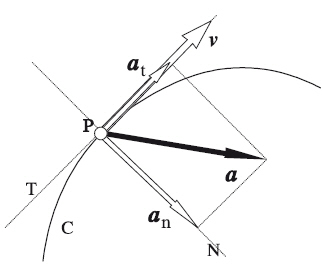# Characteristics of Acceleration in PhysicsAcceleration in dynamics and kinematics is a physical quantity that determines the change in the speed of an object. In the international system of units we must express the value of this magnitude in meters per second squared (m/s²).

In kinematics calculations it is important to establish a reference system. The speed of a person walking inside a train is not the same for an observer located inside the train as for an observer located on the platform. The reference system is the position of the observer.

## Definition of Acceleration in Physics

Acceleration is defined as the change in speed of an object in a given time interval.

It can be positive, negative, or zero, meaning that an object can be accelerating (increasing its speed), decelerating (decreasing its speed), or maintaining a constant speed. Positive acceleration occurs when an object moves faster over time, while negative acceleration occurs when its speed decreases.

## Acceleration CharacteristicsBelow are some of the key features of acceleration:

• Vector quantity: Acceleration is a vector quantity, meaning it has magnitude and direction. This implies that it can change in direction as well as magnitude, which translates into changes in speed.

• Unit of measurement: In the International System of Units (SI), the unit of measurement for acceleration is the meter per second squared (m/s²). This unit expresses how many meters per second the speed of an object changes every second.

• Relationship with force: Acceleration is related to the force acting on an object. According to Newton's second law, the net force applied to an object is equal to the product of its mass and its acceleration (F = m * a). The greater the force applied, the greater the resulting acceleration.

• Causes of acceleration: Acceleration can be caused by various forces and factors. Some of the common causes of acceleration include the application of an external force, gravity, friction, air resistance, and other physical interactions. Dynamics is the branch of physics that is responsible for studying the causes that cause an acceleration in an object. In particular, acceleration plays a fundamental role in Newton's laws.

## Types of Acceleration

Acceleration can manifest itself in various ways depending on conditions and context. Below are some of the most common types of acceleration:

### 1. Linear Acceleration

Linear acceleration refers to the change in the speed of an object in a specific direction along a straight line. It can be positive, negative or null depending on whether the speed increases, decreases or remains constant respectively.

### 2. Tangential and Centripetal AccelerationsWhen an object moves along a curved path, it experiences changes in its speed in the direction tangential to the curve. The acceleration vector of a body can have a direction different from the speed with which it is moving. This vector can be divided into two components: the tangential and the normal.

Tangential acceleration is a specific form of linear acceleration that causes a change in the speed of an object. This component has a tangential direction to the movement.

On the other hand, normal acceleration has a direction perpendicular to the movement of the body and points towards the center of the circular movement. The normal component of acceleration is what causes the change in direction, generating the curvature of the trajectory.

### 4. Angular Acceleration

Angular acceleration relates to the change in angular velocity of an object in rotating motion.

It is measured in radians per second squared (rad/s²) and can be positive or negative, depending on whether the angular velocity increases or decreases with time.

### 5. Gravitational Acceleration

Gravitational acceleration refers to the acceleration due to gravity on the Earth's surface or in the vicinity of other celestial bodies.

On Earth, this acceleration is known as the acceleration of gravity and has a value of approximately 9.81 m/s² and acts downward.

### 6. Relative Acceleration

Relative acceleration refers to the acceleration of an object relative to another moving object. It can occur when two objects move relative to each other and experience relative accelerations in different directions.

A common example of relative acceleration is the sensation of acceleration you experience when riding in a car starting from rest. From the car's perspective, you are accelerating backwards, while from your own perspective, you feel like you are accelerating forwards.

### 7. Coriolis Acceleration

Coriolis acceleration is a type of acceleration that occurs in objects moving in a non-inertial reference frame, such as the Earth's rotation.

This acceleration is responsible for effects such as the deflection of winds and ocean currents, as well as the rotation of the Earth around its axis.

Author:

Published: February 28, 2022
Last review: November 8, 2023